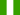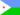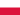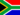Visualizzazione dei costi medi e costi marginali come pendenzaAll Modules View all topics Close topics
Modulo 1: Comprensione a Fixed, Variabile e Costi marginali in Economia

# Visualizzazione dei costi medi e costi marginali come pendenza

• Study Reminders

We'll email you at these times to remind you to study

You can set up to 7 reminders per week

#### You're all set

We'll email you at these times to remind you to study

Monday

Tuesday

Wednesday

Thursday

Friday

Saturday

Sunday

• Note di Apprendimento
• Revisione degli argomentiAmah K. 0 0 Average cost is the per unit cost. It is calculated as, total cost divided by output or quantity. Marginal cost is a firm's additional cost. It is calculated as change in total cost divided by change in output or quantity.Amah K. 0 0 Average cost is the per unit cost. It is calculated as total cost divided by output or quantity. Marginal cost is a firm's additional cost. It is calculated as change in total cost divided by change in output or quantity. Average cost curve rises intially and falls a little before remaining constant but marginal cost curve rises above average cost curve and then falls. When total cost curve is at maximum,marginal cost curve is equal to zero.Faizal A. 0 0 very nice topicKaram A. 0 0 i would like to say from my idea, When he explains the part where the programmers have less efficiency as he hires more and more programmers, is he describing the law of diminishing returns? It seems to me that some of these courses for microeconomics is out of order. For example, I personally think that this section of courses was supposed to come before the last section of courses (it certainly seems like that’s how Sal organized it) I am confused why average total cost can be greater than the marginal cost.,,Luckson N. 0 0 marginal cost is change of cost of production after adding a unit